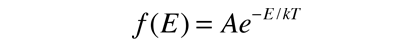# Applications of the Boltzmann Distribution

 Average particle energy Average molecular kinetic energy Maxwell speed distribution Specific heats of solids Fractional population of excited states

 Comment on statistical methods in physics
Index

Kinetic theory concepts

 HyperPhysics***** Thermodynamics R Nave
Go Back

# Average Particle Energy from Boltzmann Distribution

The Boltzmann distribution describes the distribution of energy among classical (distinguishable) particles:It can be used to evaluate the average energy per particle in the circumstance where there is no energy-dependent density of states to skew the distribution. To represent the probability for a given energy, it must be normalized to a probability of 1 :This normalized distribution function can then be used to evaluate the mean or average energy.Show

This shows that the average energy = kT when the energy is randomly distributed among the available energy states. The development of the Boltzmann distribution was under the constraints of conservation of energy and conservation of the number of particles. Note that this average energy for randomly distributed energy is not the same as the average kinetic energy.

 Applications of the Boltzmann distribution
Index

Kinetic theory concepts

 HyperPhysics***** Thermodynamics R Nave
Go Back

# Average Energy Integral: Boltzmann Distribution

The average energy integral for the distribution of energy among a collection of particles according to the Boltzmann distribution is:This integral may be evaluated using integration by parts.This technique is particularly appropriate for removing a linear term multiplying an exponential.Index

Kinetic theory concepts

 HyperPhysics***** Thermodynamics R Nave
Go Back